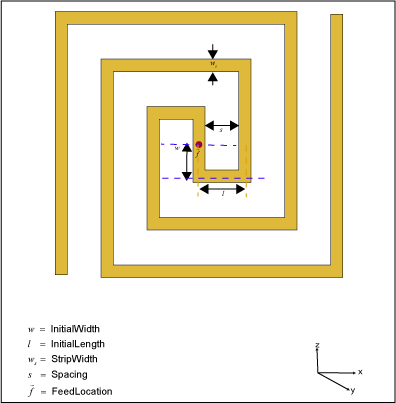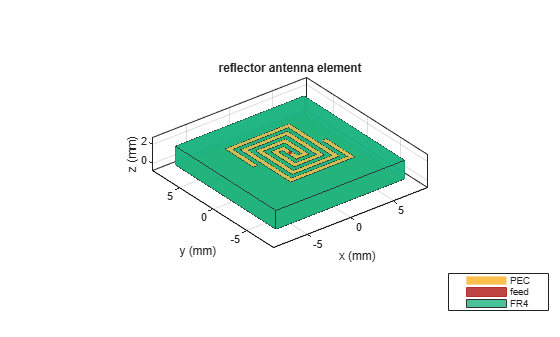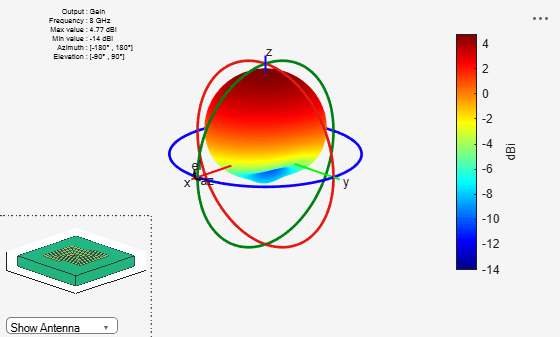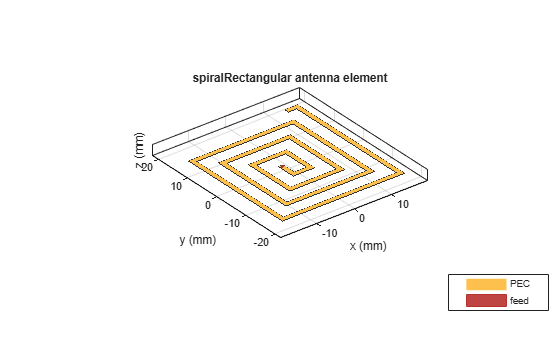# spiralRectangular

Create rectangular spiral antenna on xy-plane

Since R2020a

## Description

The `spiralRectangular` object creates a single or two-arm rectangular spiral antenna. The default rectangular spiral has two arms, is center-fed and is on the xy-plane. The default resonating frequency is 7.65 GHz.

A spiral rectangular antenna is made up of filaments. The distance between the two violet dashed lines in the diagram represents the first filament or the initial width. The distance between the two orange dashed lines in the diagram represents the second filament or the initial length.## Creation

### Syntax

``ant = spiralRectangular``
``ant = spiralRectangular(Name=Value)``

### Description

example

````ant = spiralRectangular` creates a default rectangular spiral antenna object operating at 7.65 GHz.```

example

````ant = spiralRectangular(Name=Value)` creates a rectangular spiral antenna, with additional Properties specified by one or more name-value arguments. `Name` is the property name and `Value` is the corresponding value. You can specify several name-value arguments in any order as `Name1=Value1`, `...`, `NameN=ValueN`. Properties not specified retain their default values.```

## Properties

expand all

Number of arms of the spiral, specified as `1` or `2`.

Example: `1`

Data Types: `double`

Number of turns in the spiral, specified as a scalar in meters. One turn length is taken as the length of a complete 360-degree revolution. To calculate the length of 1.25 turns, the first spiral is created up to one turn. Then the length of the second turn is scaled to the given fraction and added to the first turn length.

Example: `2`

Data Types: `double`

Length of the first filament along the y-axis from the origin to the mid-line of the strip width of the second filament, specified as a scalar in meters. `InitialWidth` is the width between the dashed violet color lines in the antenna image.

Example: `0.0050`

Data Types: `double`

Length of the second filament along the x-axis from the mid line of the first filament to half of the strip width of the third filament, specified as a scalar in meters. `InitialLength` is the width between the dashed orange color lines in the antenna image.

Example: `0.0055`

Data Types: `double`

Width of the strip, specified as a scalar in meters.

Example: `5.0050e-04`

Data Types: `double`

Spacing between turns of the spiral, specified as a scalar in meters.

Example: `0.0015`

Data Types: `double`

Direction of the spiral turns (windings), specified as `"CW"` (clockwise) or `"CCW"` (counter-clockwise).

Example: `"CW"`

Data Types: `string`

Type of the metal used as a conductor, specified as a metal material object. You can choose any metal from the `MetalCatalog` or specify a metal of your choice. For more information, see `metal`. For more information on metal conductor meshing, see Meshing.

Example: `metal("Copper")`

Lumped elements added to the antenna feed, specified as a `lumpedElement` object. You can add a load anywhere on the surface of the antenna. By default, the load is at the feed. For more information, see `lumpedElement`.

Example: `lumpedElement(Impedance=75)`

Tilt angle of the antenna in degrees, specified as a scalar or vector. For more information, see Rotate Antennas and Arrays.

Example: `90`

Example: `Tilt=[90 90]`,`TiltAxis=[0 1 0;0 1 1]` tilts the antenna at 90 degrees about the two axes defined by the vectors.

Data Types: `double`

Tilt axis of the antenna, specified as one of these values:

• Three-element vector of Cartesian coordinates in meters. In this case, each coordinate in the vector starts at the origin and lies along the specified points on the x-, y-, and z-axes.

• Two points in space, specified as a 2-by-3 matrix corresponding to two three-element vectors of Cartesian coordinates. In this case, the antenna rotates around the line joining the two points.

• `"x"`, `"y"`, or `"z"` to describe a rotation about the x-, y-, or z-axis, respectively.

Example: `[0 1 0]`

Example: `[0 0 0;0 1 0]`

Example: `"Z"`

Data Types: `double` | `string`

## Object Functions

 `show` Display antenna, array structures, shapes, or platform `axialRatio` Axial ratio of antenna `beamwidth` Beamwidth of antenna `charge` Charge distribution on antenna or array surface `current` Current distribution on antenna or array surface `design` Design prototype antenna or arrays for resonance around specified frequency or create AI-based antenna from antenna catalog objects `efficiency` Radiation efficiency of antenna `EHfields` Electric and magnetic fields of antennas or embedded electric and magnetic fields of antenna element in arrays `impedance` Input impedance of antenna or scan impedance of array `mesh` Mesh properties of metal, dielectric antenna, or array structure `meshconfig` Change meshing mode of antenna, array, custom antenna, custom array, or custom geometry `optimize` Optimize antenna or array using SADEA optimizer `pattern` Plot radiation pattern and phase of antenna or array or embedded pattern of antenna element in array `patternAzimuth` Azimuth plane radiation pattern of antenna or array `patternElevation` Elevation plane radiation pattern of antenna or array `rcs` Calculate and plot radar cross section (RCS) of platform, antenna, or array `returnLoss` Return loss of antenna or scan return loss of array `sparameters` Calculate S-parameters for antennas and antenna arrays `vswr` Voltage standing wave ratio (VSWR) of antenna or array element `rectspirallength2turns` Calculate number of turns for specified arm length in rectangular spiral antenna

## Examples

collapse all

Create and view a default rectangular spiral antenna.

`ant = spiralRectangular`
```ant = spiralRectangular with properties: NumArms: 2 NumTurns: 1.5300 InitialWidth: 0.0010 InitialLength: 0.0015 StripWidth: 4.0500e-04 Spacing: 0.0011 WindingDirection: 'CCW' Conductor: [1x1 metal] Tilt: 0 TiltAxis: [1 0 0] Load: [1x1 lumpedElement] ```
`show(ant)`Plot the radiation pattern of the antenna at the default frequency.

`pattern(ant,7.65e9)`Create a rectangular spiral antenna object with two arms and two turns.

```ant_d = spiralRectangular(NumArms=2, NumTurns=2, InitialLength=1e-3,... InitialWidth=1e-3, Spacing=0.5e-3, StripWidth=0.5e-3);```

Back the spiral using a reflector antenna object.

```r = reflector(Exciter=ant_d, GroundPlaneLength=15e-3, GroundPlaneWidth=... 15e-3, Spacing=2e-3, Substrate=dielectric("FR4")); figure show(r);```Plot the radiation pattern of the antenna at the specified frequency.

```figure pattern(r,8e9);```Create a single arm rectangular spiral antenna with a total arm length of 291 mm.

```ant = spiralRectangular(NumArms=1, NumTurns=3, InitialLength=4.5e-3,... InitialWidth=4.5e-3, Spacing=3.3e-3, StripWidth=1.2e-3); nT = rectspirallength2turns(ant,291e-3); ant.NumTurns = nT; figure show(ant) ```Nakano, H., H. Yasui, and J. Yamauchi. “Numerical Analysis of Two-Arm Spiral Antennas Printed on a Finite-Size Dielectric Substrate.” IEEE Transactions on Antennas and Propagation 50, no. 3 (March 2002): 362–70. https://doi.org/10.1109/8.999628.

 Nakano, H., J. Eto, Y. Okabe, and J. Yamauchi. “Tilted- and Axial-Beam Formation by a Single-Arm Rectangular Spiral Antenna with Compact Dielectric Substrate and Conducting Plane.” IEEE Transactions on Antennas and Propagation 50, no. 1 (January 2002): 17–24. https://doi.org/10.1109/8.992557.